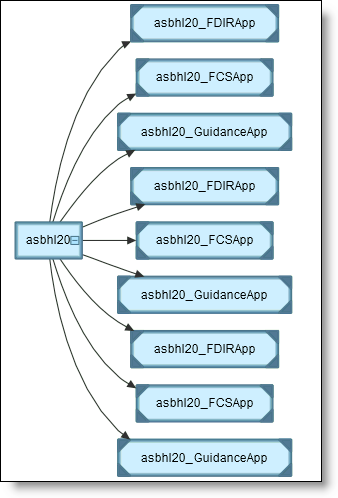# How Many Blocks are in that Model?

My colleague Sam and I wanted to find an example model with about 10,000 blocks.  I suggested the HL-20 model from the Aerospace Blockset, asbhl20.mdl.  Sam decided to measure the total number of blocks by running sldiagnostics:

>> [t,s] = sldiagnostics('asbhl20','CountBlocks'); s(1) ans =     isMask: 0       type: 'asbhl20 Total blocks'      count: 5700

Sam quickly sent me a message saying that the HL-20 model only had 5700 blocks.  What gives?

What is SLDIAGNOSTICS?

First, let us discuss a little history of sldiagnostics.  Over the years, Simulink developers have worked with many customers to learn about their modeling styles and assist in debugging problems.  Unfortunately some customers are unable to share their models because they might contain proprietary information.  The solution these developers created were a series of scripts that would quickly gather information about a model without any model algorithm information. These collected scripts then became the sldiagnostics function.  Because the output of these scripts was generally devoid of any proprietary data, even customers who could not share their models were able to provide the diagnostic information instead.

Most of the checks done by sldiagnostics help you gather an understanding of the model as a whole.  This is a great help in learning about models rather than having to browse through every system one-by-one.

CountBlocks

The diagnostic information Sam gathered was the output of CountBlocks.  Here is a part of the text report for the HL-20 model.

Finished counting blocks in 'asbhl20'. Found 5700 blocks.            asbhl20 Total blocks :  5700                           Abs :    16                    ActionPort :    11                     Assertion :     2                          Bias :     6                    BusCreator :    46                   BusSelector :   126             <snip>                        Inport :  1175                  InportShadow :    49                    Integrator :    43             Interpolation_n-D :     1                         Logic :     6                        Lookup :     6                    Lookup_n-D :     6                          Math :    59                        Memory :     4                         Merge :     4                        MinMax :     4                ModelReference :     9               MultiPortSwitch :     1                           Mux :   141                       Outport :   669             <snip>

HL-20 Includes Model Reference

The HL-20 model includes 9 ModelReference blocks.  (I introduced some of the basics of model reference a previous post.)  You can see the hierarchy of the model in the Dependency Viewer instance view.When I think about a model, I imagine the net collected functionality represented by it.  I think the number of blocks used to create that model is a simple measure for how much functionality/algorithm there is.  Model reference blocks contain the functionality of the entire model that they reference, however, sldiagnostics counts ever Model Reference block as only one block.

The measure I am really looking for is the subsystem equivalent implementation of all the blocks in the model.  To get this for the HL-20 model, you actually need to count the blocks inside each model referenced and multiply by the number of instances.  I wrote a MATLAB function to do this:

function totalBlocks = mdlrefCountBlocks(mdl) % mdlrefCountBlocks Count the subsystem equivalent number of blocks % in a model reference hierarchy.   % Copyright 2009 The MathWorks, Inc   %% Open the model open_system(mdl)   %% Get instance information [mDep,mInst] = find_mdlrefs(mdl); % Open dependent models for i = 1:length(mDep)     load_system(mDep{i}) end   %% Count the number of instances of each dependency mCount = zeros(size(mDep)); mCount(end) = 1; % Last element is the top model, only one instance for i = 1:length(mInst)     mod = get_param(mInst{i},'ModelName');     mCount = mCount + strcmp(mod,mDep); end %% for i = 1:length(mDep)     disp([num2str(mCount(i)) ' instances of ' mDep{i}]) end disp(' ')   %% Loop over dependencies, get number of blocks s = cell(size(mDep)); for i = 1:length(mDep)     [t,s{i}] = sldiagnostics(mDep{i},'CountBlocks');     disp([mDep{i} ' has ' num2str(s{i}(1).count) ' blocks']) end   %% Multiply number of blocks, times model count, add to total totalBlocks = 0; for i = 1:length(mDep)     totalBlocks = totalBlocks + s{i}(1).count * mCount(i); end   disp(' ') disp(['Total blocks: ' num2str(totalBlocks)])

Total Blocks

Here are the results of running this function:

>> total = mdlrefCountBlocks('asbhl20'); 3 instances of asbhl20_FCSApp 3 instances of asbhl20_FDIRApp 3 instances of asbhl20_GuidanceApp 1 instances of asbhl20   asbhl20_FCSApp has 115 blocks asbhl20_FDIRApp has 1248 blocks asbhl20_GuidanceApp has 388 blocks asbhl20 has 5700 blocks   Total blocks: 10953